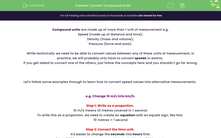# Convert Compound Units

In this worksheet, students will practise converting compound speed values into alternative units to find equivalents.Key stage:  KS 4

Year:  GCSE

GCSE Subjects:   Maths

GCSE Boards:   AQA, Eduqas, Pearson Edexcel, OCR,

Popular topics:   Percentages worksheets, Ratio worksheets

Difficulty level:#### Worksheet Overview

Compound units are made up of more than 1 unit of measurement e.g.

Speed (made up of distance and time);

Density (mass and volume);

Pressure (force and area).

While technically we need to be able to convert values between any of these units of measurement, in practice, we will probably only have to convert speeds in exams.

If you get asked to convert one of the others, just follow the concepts here and you shouldn't go far wrong.

Let's follow some examples through to learn how to convert speed values into alternative measurements.

e.g. Change 10 m/s into km/h.

Step 1: Write as a proportion.

10 m/s means 10 metres covered in 1 second.

To write this as a proportion, we need to create an equation with an equals sign, like this:

10 metres = 1 second

Step 2: Convert the time unit.

It's easier to change the seconds into hours first.

1 hr = 3600 seconds, so we can multiply both sides by 3600 to get:

36000 metres = 3600 seconds

36000 metres = 1 hour

Step 3: Convert the speed unit.

We know that 1 km = 1000 m.

We can now change the distance travelled into kilometres by dividing by 1000 to reach:

36 km = 1 hour

Since we now have our units expressed in kilometres and hours, we can say that 10 m/s = 36 km/h.

Let's try another to check that we have this process clear.

e.g. Change 20 km/h into m/s.

Step 1: Write as a proportion.

20 km/hr means 20 kilometres covered in 1 hour.

20 kilometres = 1 hour

Step 2: Convert both units to their equivalents.

We know that 1 hr = 3600 seconds and 1 km = 1000, so we can convert both sides into these alternative units:

20 kilometres = 1 hour  -->  20000 m = 3600 seconds

Step 3: Convert the time to 1 second.

We currently know how many metres we travel in 3600 seconds, but we want to know how many we travel in just 1 second.

To find this, we need to divide both sides by 3600:

5.56 m = 1 second

Since we now have our expressed in metres and seconds, we can say that 20 km/h = 5.56 m/s.

In this activity, we will convert compound speed values into alternative units to find equivalents using variations of the method shown above.

### What is EdPlace?

We're your National Curriculum aligned online education content provider helping each child succeed in English, maths and science from year 1 to GCSE. With an EdPlace account you’ll be able to track and measure progress, helping each child achieve their best. We build confidence and attainment by personalising each child’s learning at a level that suits them.

Get started#### Popular Maths topics

••••